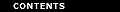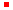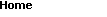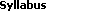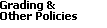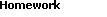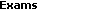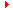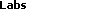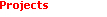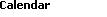The trajectory of light as an example of the shortest path

The minimal problem
Light is known to propagate along straight lines in a uniform medium. Going from one point to another, it chooses the shortest path. In this lab, you will answer the question: What is the trajectory of light that starts at point P and passes through point Q after being reflected at some unknown point R on mirror M in between?

To specify the point R, we apply the principle of the shortest distance. This principle requires that the sum

PR + RQ
be minimal. This minimum requirement alone will specify the location of R on the mirror.

First solution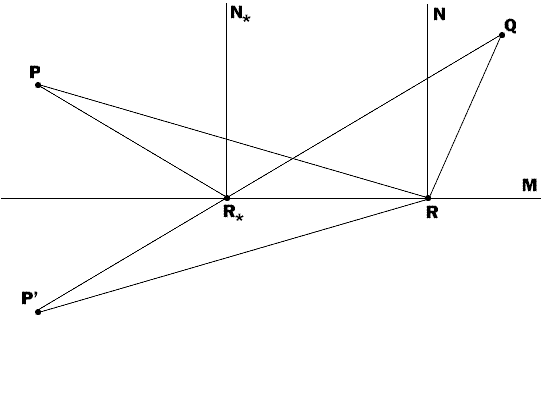We know from daily experience that an observer at Q sees the reflected light coming from an imiginary reflected source P' seemingly located behind the mirror. The trajectory of light connecting P' with Q should be the shortest possible path between these points, i.e. it should be the straight line P'Q. This line intersects with the mirror M at point R*. Since PR* = P'R*, we have
PR* + R*Q = P'R* + R*Q = P'Q.
This is the shortest distance because P'Q is a straight line. To prove this, assume the contrary, i.e. that the reflection occurs at some point R rather than at R*. For the triangle P'QR we have the inequality
P'R + RQ > P'Q = P'R* + R*Q.
This inequality shows that the straight line P'Q yields the shortest possible distance.

An elementary geometric analysis shows that if N*R* is the normal to M at R*, then the angle of incidencePR*N* is equal to the angle of the reflectionN*R*QPR*N* =N*R*Q,
and this equality of angles fixes the choice R* of R. For any other point R on the mirror, the equality of the relevant angles will not occurPRN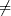NRQ.

Second solution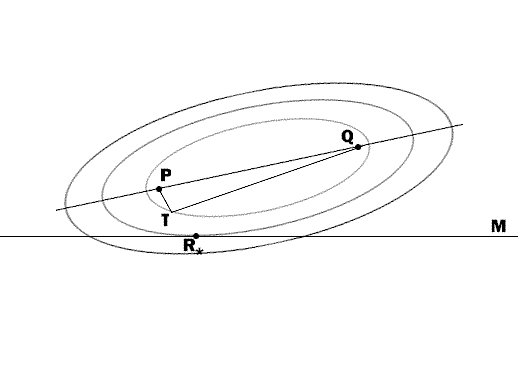Introduce the function(T) = PT + TQ
defined as the sum of distances from two fixed points P and Q to a variable point T. Let us fix a positive number d > PQ and consider the locus of points T having the same value of(T) equal to d:(T) = d.

All such points belong to an ellipse with foci P and Q. If we choose a number d1 > d, then the equation(T) = d1 will define a larger ellipse, confocal with the previous one. If we picked other values of d and plotted the corresponding ellipses, we would come up with a series of level curves(T) = const; this chart being a family of confocal ellipses with focal points P and Q.

Some of these ellipses intersect with the mirror M at two points, some do not intersect it at all, and only one ellipse has one common point with the mirror. This selected point is the required point R* of reflection, and the relevant ellipse specifies the minimum distance PR* + R*Q.

Problems

1. Let (xP, yP) be the coordinates of P, (xQ, yQ) be the coordinates of Q, and (x, y) be the coordinates of R. Without lack of generality, we may assume that the mirror lies along the x-axis (y = 0). The sum PR + RQ can be computed
PR + RQ = sqrt((xP - x)2 + yP2) + sqrt((xQ - x)2 + yQ2).
This sum (which is a function of x) should be minimal. Let x* be the value of x minimizing this sum and R*(x*, 0) be the corresponding point on the mirror.

Show by direct calculation that

 min(PR + RQ) = (PR + RQ)x = x* = PR* + R*Q = P'R* + R*Q = P'Q = sqrt((xQ - xP)2 + (yQ + yP)2)

2. Comparing the first and second solutions, demonstrate the following property of an ellipse: Two straight lines connecting the focal points of an ellipse with an arbitrary point R on its periphery make equal angles with the tangent to the ellipse at point R. Your solution does not need to contain any formulas. A well-worded description will suffice.

3. Based on the second solution, suggest, in words, a procedure for finding the point of light reflection from a curvilinear mirror of arbitrary shape.

4. Three towns A, B, and C want to build three roads towards a common transportation center. They want to choose the location R of this center so as to minimize the total length of the roads and, hence, the construction costs. Formulate this minima problem and solve it by applying a procedure similar to the one described above in the second solution. Use the result of Problem 2 to give a complete descriptive characterization of the desired location R.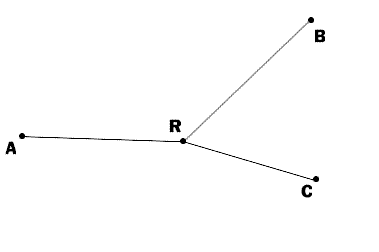Back to the top

Created by Henry Fink
Last updated: Sunday, September 28, 1997